## Biquad Active Filter

### Introduction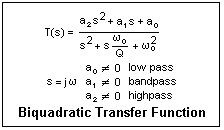Operational amplifiers can be used to realize a linear system with an arbitrary biquadratic transfer function, as shown at the right. The complex variable s = jω, where ω = 2πf. This function is the ratio of two quadratic expressions in s. The denominator specifies the characteristic frequency ω0 and the Q-factor Q. The three arbitrary complex constants a0, a1 and a2 specify the filter properties in terms of the basic filter types of high pass, bandpass and lowpass, which occur when only one of the constants is nonzero. Combinations of these types create further filter types, such as notch and allpass filters.

The parameter Q has an easily appreciated meaning in the case of the bandpass filter. The gain is maximum at the angular frequency ω0 and falls off at lower and higher frequencies. Near the centre frequency, the denominator can be written as (ω0 + ω)(ω0 - ω) + jωω0/Q, or approximately as 2ω0Δω + jω0ω/Q. The magnitude of the transfer function will be down by 1/√2 when these two terms are equal in magnitude, or Δω = ω0/2Q. Therefore, the total width of the peak at half maximum power (the square of this) is ω0/Q.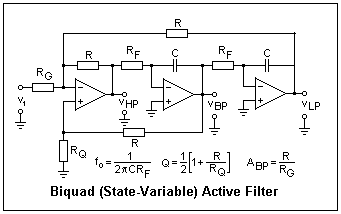A biquad transfer function can be realized straightforwardly with a pair of integrators in series, each introducing a factor 1/s, with their outputs added to the input signal in a summer, as illustrated in the circuit at the left. This circuit looks complex at first, but if you carry out a circuit analysis its operation will become clear. Assume that the signal at the output of the summer, the leftmost operational amplifier, is x. Then find the signals that are added at the summer in terms of x, and finally solve for x in terms of the input signal v1. You will find that the result is in the form of a high pass transfer function. The outputs of the following two amplifiers will be a bandpass function and a lowpass function. This amplifier realizes the three basic amplifier types, which can then be combined as desired.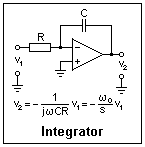The effect of an integrator stage is illustrated at the right, to help in the analysis. The parameters f0, Q and the bandpass gain at the centre frequency are given in terms of the circuit parameters in the figure. These expressions are sufficient for the design of a filter. In any case, resistances should be 5k or greater to avoid overloading the operatonal amplifiers. The frequency-determining values of RF and C must be carefully matched if a high Q is to be realized.

A circuit I tested used the following values: C = 0.01μF, RF = 16k, RG = R = 10k, RQ = 1k (not an op-amp load). The amplifiers were LM833 dual op-amps, which are intended for audio applications. This circuit should have f0 = 1kHz, Q = 5.5 and ABP = 1. I applied a 2V p-p input signal whose frequency was measured by a counter. The input and ouput were displayed on an oscilloscope. The HP, LP and BP outputs should all be examined as the frequency is varied to either side of 1 kHz. Note the peaks on the HP and LP responses, and the phase relation between input and output at the peaks. Estimate the Q from the BP response by finding the 1/&root;2 points.

Some references show the input applied to the noninverting input of the summer. Of course, this changes the sign of the gains, but otherwise seems to work in about the same way. An accurate analysis is more difficult for this configuration. It does not seem to have any advantage over input to the inverting input.

### Another Biquad FilterA different biquad filter is shown at the left. In this circuit, the first integrator is also used as the summer. One might think that in this case only two op-amps would be required, but unfortunately a signal sign change is necessary, so a unity-gain inverting amplifier is included. The inverting amplifier and the second integrator may be in either order. A drawback is that the highpass response is not available, only the bandpass and lowpass functions.

It is instructive to analyze this circuit, which is straightforward since there is a virtual ground node at each amplifier. The feedback impedance of the first integrator is RB/(1 + jωRBC), which can be used in the usual expressions for the gain of an inverting amplifier. Some algebra is necessary, but the appropriate biquad transfer function is obtained.

RF and C determine the centre frequency ω0. The value of R is unimportant; 10k is a usual value. RB determines Q = RB/RF and the bandpass gain ABP = RB/RG. The bandwith Δf = fo/Q = 1/2πCRB depends only on RB, which can be said to set the bandwidth instead of Q, independent of frequency. RF can be varied to change the centre frequency, perhaps using ganged rheostats. However, to maintain a high Q the resistances must track very accurately.

For a test circuit, I used RF = 16k and C = 0.01μF as for the previous circuit. R was 10k, and RB = RG = 33k. These values gave fo = 995 Hz, Δf = 483 Hz, Q = 2.06 and ABP = 1.

### Notch Filter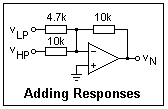If you make a weighted sum of the lowpass and highpass functions of the first biquad filter with the circuit shown at the right, the result will be a notch filter with a notch at about 1.46 kHz. The gain at low frequencies will be +1.0, and at high frequencies about +0.5. The response goes through a maximum with gain about 2 at 917 Hz, while the phase shift is 90° at the corner frequency of 1.031 Hz.

If the weighted sum is vHP + AvLP, it is easy to show that the notch frequency is given by fN/fo = √A. The notch in this case is just higher than the corner frequency. If A < 1, then the notch is lower than the corner frequency. The numerator of the transfer function is ω2 - Aωo2, and the notch occurs when this expression becomes zero.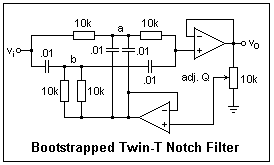It is instructive to compare the biquad notch filter with the remarkable twin-T notch filter, which is a passive filter with a transmission zero in spite of not including inductance. The circuit shown uses op-amps to isolate the filter from its load, as well as to "bootstrap" it so the effective Q can be varied. The circuit I tested was constructed from random components and gave a reasonable zero, but not one as good as the biquad filter provided. For a good zero, the components must be very closely matched, using at least 1% resistors and capacitors. This circuit gives a zero at 1591 Hz. The general formula is fn = 1/2πRC.

With the resistors and capacitors at the base of the T grounded, the effective Q is about 1/4. If the amount of bootstrapping is increased, Q increases, but the effect of mismatched components is greatly magnified, and the zero becomes quite poor. To analyze this circuit, write nodal equations for nodes a and b. If x = ωRC, we find vo = vi[(1 - x2)/(1 + 4jx - x2)], which is a biquadratic response with Q = 1/4. The gain approaches 1 for low frequencies, and -1 at high frequencies.

### References

You may want to review the articles on Time and Frequency Domains (8), Filters (16), and LC Filters (33).

A. S. Sedra and K. C. Smith, Microelectronic Circuits, 2nd ed. (New York: Holt, Rinehart and Winston, 1987), pp. 784-803.

P. Horowitz and W. Hill, the Art of Electronics, 2nd ed. (Cambridge: Cambridge Univ. Press, 1989, pp. 276-279.

National Semiconductor, data sheet for LM833N.